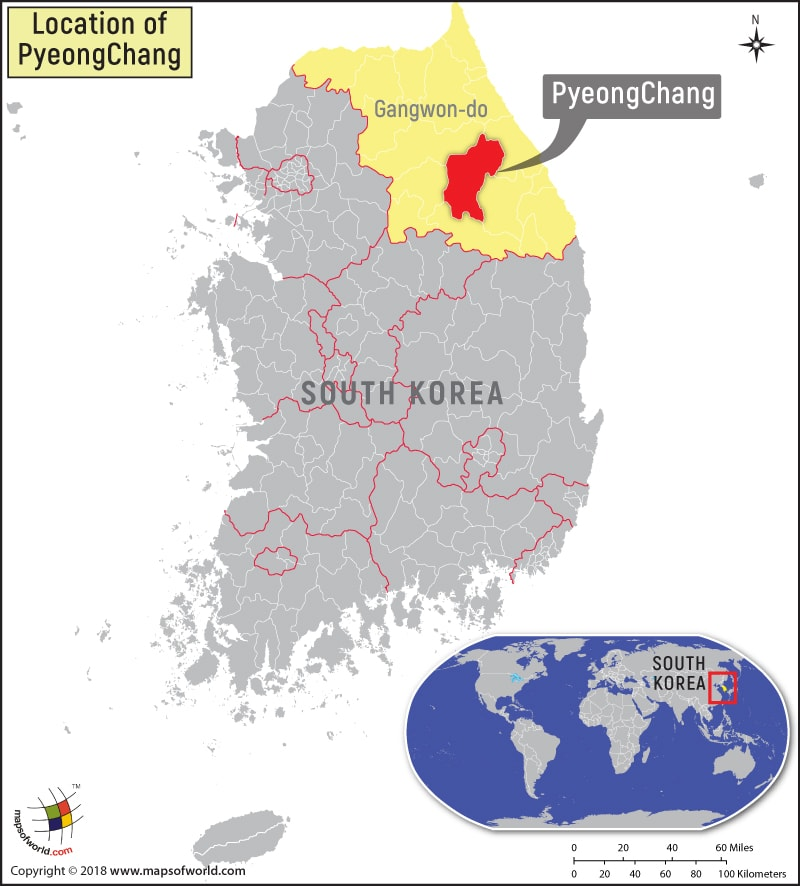﻿ Where is PyeongChang Located? Location of PyeongChang, South Korea

# Where is PyeongChang LocatedDescription :  Map showing location of PyeongChang in South Korea. Disclaimer

 Close Disclaimer : All efforts have been made to make this image accurate. However Compare Infobase Limited,its directors and employees do not own any responsibility for the correctness or authenticity of the same.

Search For Latitude & Longitude Map

By Country

Or

Latitude

Longitude

Or

Area

* Put South & West values with minus ( - ) sign.

Where is PyeongChang?

The 2018 Winter Olympics will be hosted in PyeongChang, which is located in Gangwon province, South Korea. The county, which has many Buddhist temples, is situated in the Taebaek Mountains region. The county is located at a distance of 110 miles from the South Korean capital of Seoul. The county covers an area of 565.12 square miles and as of 2013, it comprised a population of 43,666 people.

The county is popular among trekkers as around 84 percent of its territory has mountains. Though the summers are warm, winters are long and snowy. Two major ski resorts are located at this popular tourist destination. These are the Alpensia ski resort and the Yongpyong Resort. Both these resorts will host the 2018 Winter Olympics. The technical alpine skiing events will be held at the Yongpyong Resort. The resort has 31 slopes and is the largest in Korea. Most of the snow events would be played at the Alpensia, which has six slopes. With the completion of the Gyeonggang Line, which is being built for the 2018 Winter Olympics, it would take just 80 minutes to reach PyeongChang from Seoul.

City Name PyeongChang
Continent Asia
Country South Korea
Region Gangwon
Area 565.12 sq mi
Population 43,666 (2013)
Lat Long 37.3705? N, 128.3900? E
Avg Temperature High 52.7 F, Low 35.6 F
Calling Code +82 33
Time Zone UTC+09:00
Airport Yangyang International Airport
Currency South Korean Won

 Location Maps of Cities in South Korea
 Where is Aewol li Where is Andong Where is Ansan Where is Ansong Where is Anyang Where is Asan Where is Cheju Where is Chinhae Where is Chinju Where is Chinyong Where is Chonju Where is Gwangju Where is Haenam Where is Hanam Where is Hayang Where is Hongsong Where is Hunghae Where is Hwado Where is Hwasun Where is Hwawon Where is Iksan Where is Incheon Where is Incheon Where is Kanghwa Where is Kangnung Where is Kijang Where is Kimhae Where is Kimje Where is Koje Where is Kongju Where is Kosong Where is Kumi Where is Kumsan Where is Kunsan Where is Kuri Where is Kwangju Where is Kwangmyong Where is Kwangyang Where is Kyongju Where is Kyongsan Where is Masan Where is Miryang Where is Munsan Where is Naeso Where is Naju Where is Namwon Where is Namyangju Where is Nonsan Where is Osan Where is Polgyo Where is Poryong Where is Puan Where is Pubal Where is Pusan Where is Puyo Where is PyeongChang Where is Sangju Where is Seoul Where is Sinhyon Where is Songhwan Where is Songnam Where is Sonsan Where is Sosan Where is Suwon Where is Taebaek Where is Taegu Where is Taejon Where is Taesan Where is Tangjin Where is Tonghae Where is Uijongbu Where is Ulsan Where is Ungsang Where is Wabu Where is Waegwan Where is Wonju Where is Yangsan Where is Yesan Where is Yoju Where is Yongdong Where is Yonggwang Where is Yongju Where is Yongwol Where is Yonil Where is Yonmu Where is Yosu